• ## Outreach

Molecular Dynamics

Atomic positions obey Newton's second lawwhere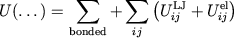The evaluation of the forcesis very time consuming. To speed up these calculations, NAMD uses:Spatial Decomposition

NAMD divides the simulation space into rectangular regions called patches. Each patch is responsible for updating the coordinates of the atoms contained in its region of space. Patch dimensions are chosen to be greater than the cutoff radius for non-bonded interactions, which eliminates the need for communication between non-adjacent patches. A cluster of adjacent patches is assigned to each processor. This design reduces the amount of interprocessor communication, enabling scalable parallelism.

particles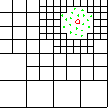level 3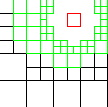level 4level 2Enhanced Parallel Fast Multipole Algorithm
(Board et al. 1995)

The effect of a charged particle is represented directly at a short length scale. At longer length scales its effect is pooled with other charges and represented by a multipole expansion, which is transfered to distant cubes and expressed as Taylor expansions.

Verlet-I / r-RESPA / Impulse MTS Method

Forces are approximated by a sequence of impulses: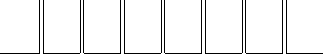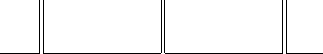Partitioning the potential energy as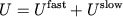allows the more numerous (long-range) slow interactions to be computed less frequently.consists of long-range electrostatics,slow part fast part

Mollified Impulse Method

The Impluse MTS method is unstable unless:

 slow-forcehalf the period of the highest frequency fast-force motion

This is overcome by the Mollified Impulse method (MOLLY).

Define vibration-averaged positionsand replacebyin the Impulse MTS method.projection in configuration space of positions onto equilibrium manifold forSome graphical MOLLY results:total energy vs. time for6.25 fs percent relative drift vs. time

Timesteps for Different Interactions

For a harmonic oscillator:

 choosing1/12 gives frequency error1.14%

The generalized period for an interaction iswhere the Hessian of an interaction is the matrix of 2nd derivatives of its potential.

Examples of:

 period(s)Normal mode analysis of 125 water molecules 9.9, 10.1 fs 0.8 fs 18.9 fs 1.6 fs

 total time worst caseSimulations measuring the generalized period of the Lennard-Jones potential for 125 water molecules at 300 K 10 fs 2.3 fs 100 fs 2.0 fs 1000 fs 1.7 fs

Avoiding Distance Calculations

By construction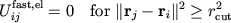This is true for most atom pairs.

To avoid most redundant distance calculations, monitor atom movement after patch assignment. Chooseto be the maximum distance traveled by an atom.Multiple Grid N-body Solvers

These are interpolation-based solvers which utilize a splitting of the potential into short-range and smooth long-range parts:

• short-range contribution: direct calculation using spatial hashing
• long-range contribution: approximate fast matrix-vector product on a grid using a multilevel method

The motivation is to be faster than the fast multipole algorithm:

• relative simplicity of the algorithm enables the avoidance of black box overhead
• continuity of forces results in stable time stepping
• continuity of force decomposition results in stable multiple time stepping

The following tables compare an initial implementation of the multiple grid N-body solver with DPMTA (Board et al.), a fast multipole implementation. The test system was a 60 Angstrom box of water containing a total of 20544 atoms. The timings were performed after compiling both codes with the cc -fast option on a Sun Ultra-60.

 grid spacing (Angstroms) average force error (%) maximum force error (%) energy error (%) time (seconds) Multiple grid N-body solver (cutoff radius = 8 Angstroms) 4.36 0.29 1.32 0.0013 1.87 2.77 0.17 0.67 0.0024 2.95 2.03 0.14 0.58 0.0017 8.15

 number of terms average force error (%) maximum force error (%) energy error (%) time (seconds) DPMTA, fast multipole implementation (theta = 0.75) 4 0.30 1.83 0.0004 6.49 8 0.02 0.25 0.0002 8.42

Fast Matrix-Vector Product on a Grid

The electrostatic potential energy due to N charged particles iswhere the set of exclusions includes all self-interactions and typically any pairs of atoms involved in some bonded interaction.

To compute the long-range contribution, we need to form the product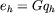which is obtained by approximating the smooth part of G on a grid of spacing h.

A fast way to do this is to approximate the smooth part of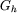on a coarser grid with spacing 2h. Using superscript k to represent a grid with spacingthe computation through L grid levels is represented schematically: